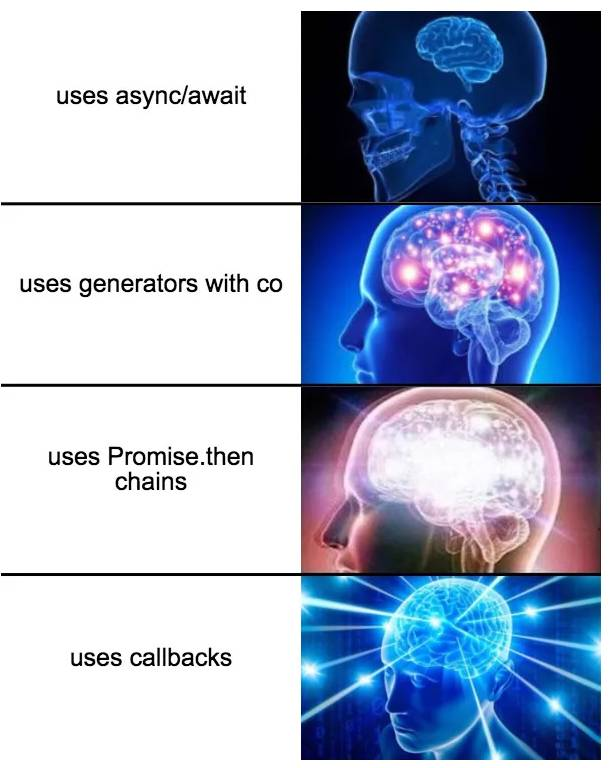02 August 2017

## 前言## 关于异步

``````var a = 1;
var b = 1;

function foo() {
a = b + 3;
console.log(a);
}

function bar() {
b = a * 2;
}

ajax("url1", foo);
ajax("url2", bar);
``````

## 异步编程方法

js 中的异步编程共有下面这些方法，好吧以前我就知道前两个，其中事件监听和观察者有些类似，下面我们将分别介绍

• 回调函数
• 事件监听
• 观察者
• Promise
• Generator
• async/await## 回调函数

callback 是我们最熟悉的方式，上面提到的动画的例子，用 callback 实现代码如下

``````moveTo(100, 0, function () {
moveTo(200, 0, function () {
moveTo(300, 0, function () {
moveTo(400, 0, function () {
// 无限不循环
});
});
});
});
``````

``````function moveTo(x = 0, y = 0, cb = function () {}) {
move(".box").x(x).y(y).end(cb);
}
``````

• 违反直觉
• 错误追踪
• 模拟同步
• 回调地狱
• 并发执行
• 信任问题（多次调用）

jquery 的 ajax 就是典型的分离回调

``````function success(data) {
console.log(data);
}
function error(err) {
console.error(err);
}
\$.ajax({}, success, error);
``````

Node 采用的是 first error，所有系统异步接口第一个参数都是 error 对象

``````function callback(err, data) {
if (err) {
// 出错
return;
}
// 成功
console.log(data);
}
async("url", callback);
``````

``````for (let i = 0; i < arr.length; ++i) {
arr[i] = sync(arr[i]);
}
``````

``````(function next(i, len, callback) {
if (i < len) {
async(arr[i], function (value) {
arr[i] = value;
next(i + 1, len, callback);
});
} else {
callback();
}
})(0, arr.length, function () {
// All array items have processed.
});
``````

## 观察者

``````sub("1", function () {
moveTo(100, 0, function () {
pub("2");
});
});

sub("2", function () {
moveTo(200, 0, function () {
pub("3");
});
});

sub("3", function () {
moveTo(300, 0, function () {
pub("4");
});
});

// 无限不循环
``````

pub 和 sub 函数的是怎么实现的呢？最简单的实现如下

``````let eventMap = {};
function pub(msg, ...rest) {
eventMap[msg] &&
eventMap[msg].forEach((cb) => {
cb(...rest);
});
}

function sub(msg, cb) {
eventMap[msg] = eventMap[msg] || [];
eventMap[msg].push(cb);
}
``````

## Promise

``````moveTo(100, 0)
.then(function () {
return moveTo(200, 0);
})
.then(function () {
return moveTo(300, 0);
})
.then(function () {
return moveTo(400, 0);
})
.then(function () {
// 无限不循环
});
``````

``````function moveTo(x = 0, y = 0) {
return new Promise(function (resolve, reject) {
move(".box").x(x).y(y).end(resolve);
});
}
``````

Promise 其实是一种控制反转，举个例子，就是原来我们要给异步函数传入一个回调函数，现在变成了异步函数返回一个 Promise 对象，堪称神来之笔，而 Promise 就是实现这种反转的工具，Promise 是一个双方约定的契约（规范）

Promise 解决了回调的一些问题，但并没有全部解决，比如 Promise 有很好的错误追踪，避免了回调地狱，对并发执行很友好，因为 Promise 只决议一次，就很好的解决了信任问题

``````var chain = Promise.resolve();
for (let i = 0; i < arr.length; ++i) {
chain = chain.then(() => async(arr[i]).then((x) => (arr[i] = x)));
}
``````

## Generator

Generator 是一个革命性特性，es2015(es6)中引入，让原本必须一次执行完毕函数，现在可以在中间暂停，并在下次继续执行，这就让 js 可以模拟协程的概念

``````function* gen() {
yield moveTo(100, 0);
yield moveTo(200, 0);
yield moveTo(300, 0);
yield moveTo(400, 0);

// 无限不循环
}

run(gen);
``````

moveTo 函数如下

``````function moveTo(x = 0, y = 0) {
return new Promise(function (resolve, reject) {
move(".box").x(x).y(y).end(resolve);
});
}
``````

``````function run(fn) {
let gen = fn();

function next(data) {
var res = gen.next(data);
if (res.done) return res.value;

res.value.then(function (data) {
next(data);
});
}

next();
}
``````

Promise 那一节，我说如果没有 Promise 就可能没有 generator，而不是必须呢？因为 generator 实现异步除了基于 Promise，还可以基于 thunk 函数，感兴趣的你自行查阅吧，祝好

## async/await

``````async function run() {
await moveTo(100, 0);
await moveTo(200, 0);
await moveTo(300, 0);
await moveTo(400, 0);

// 无限不循环
}

run();
``````

moveTo 函数如下

``````async function moveTo(x = 0, y = 0) {
await new Promise(function (resolve, reject) {
move(".box").x(x).y(y).end(resolve);
});
}
``````

## 总结xiDaiDai on 04 Aug 2017

zanjozhi on 04 Aug 2017muyudou on 07 Mar 2018

generator的看一遍忘一遍，看见async后，只想学这个。。。